## 第15章动量守恒定律及其应用

•  2018/11/30
•  180 次
•  897 K

1．篮球运动员通常伸出双手迎接传来的篮球。接球时，两手随球迅速收缩至胸前。这样做可以（    ）

A．减小球对手的冲量                  B．减小球对手的冲击力

C．减小球的动量变化量              D．减小球的动能变化量

【答案】

B

【解析】

2．一个质量是 0.05 kg 的网球，以 20 m/s 的水平速度飞向球拍，被球拍打击后，反向水平飞回，飞回的速度大小也是 20 m/s。设网球被打击前的动量为 p，被打击后的动量为 pʹ，取打击后飞回的方向为正方向，关于网球动量变化的下列计算式，正确的是（    ）

Apʹ − p = 1 kg·m/s −（− 1 kg·m/s） = 2 kg·m/s

Bppʹ = − 1 kg·m/s − 1 kg·m/s = − 2 kg·m/s

Cpʹ − p = − 1 kg·m/s − 1 kg·m/s = − 2 kg·m/s

Dppʹ = 1 kg·m/s − 1 kg·m/s = 0

【答案】

A

【解析】

3．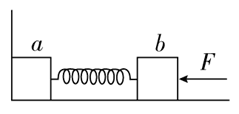木块 a 和 b 用一根轻弹簧连起来，放在光滑水平面上，a 紧靠在墙壁上。在 b 上施加向左的水平力使弹簧压缩，如图所示。当撤去外力后，下列说法中正确的是（    ）

A．a 未离开墙壁前，a 和 b 系统的动量守恒

B．a 未离开墙壁前，a 和 b 系统的动量不守恒

C．a 离开墙后，a 和 b 系统的动量守恒

D．a 离开墙后，a 和 b 系统的动量不守恒

【答案】

BC

【解析】

a 未离开墙壁前，受到墙的作用力，系统所受外力不为零，所以 a 和 b 系统的动量不守恒；a 离开墙后，a 和 b 系统水平方向不受外力，系统动量守恒。

4．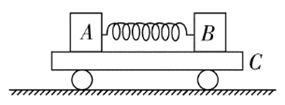如图所示，A、B 两物体质量之比 mAmB = 3∶2，原来静止在平板小车 C 上，A、B 间有一根被压缩的弹簧，水平地面光滑。当弹簧突然释放后，则（     ）

A．若 A、B 与平板车上表面间的动摩擦因数相同，A、B 组成的系统动量守恒

B．若 A、B 与平板车上表面间的动摩擦因数相同，A、B、C 组成的系统动量守恒

C．若 A、B 所受的摩擦力大小相等，A、B 组成的系统动量守恒

D．若 A、B 所受的摩擦力大小相等，A、B、C 组成的系统动量守恒

【答案】

BCD

【解析】

5．甲、乙两名滑冰运动员沿同一直线相向运动，速度大小分别为 3 m/s 和 1 m/s，迎面碰撞后（正碰）甲、乙两人反向运动，速度大小均为 2 m/s，则甲、乙两人质量之比为（    ）

A．2∶3         B．2∶5          C．3∶5          D．5∶3

【答案】

C

【解析】

mv + mv = mvʹ+ mvʹ，

6．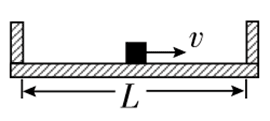质量为 M、内壁间距为 L 的箱子静止于光滑的水平面上，箱子中间有一质量为 m 的小物块，小物块与箱子底板间的动摩擦因数为 μ。初始时小物块停在箱子正中间，如图所示。现给小物块一水平向右的初速度 v，小物块与箱壁碰撞 N 次后恰又回到箱子正中间，并与箱子保持相对静止。设碰撞都是弹性的，则整个过程中，系统损失的动能为（    ）

A．$$\frac{1}{2}$$mv2               B．$$\frac{{mM}}{{2(m + M)}}$$v2             C．$$\frac{1}{2}$$NμmgL         D．NμmgL

【答案】

BD

【解析】

7．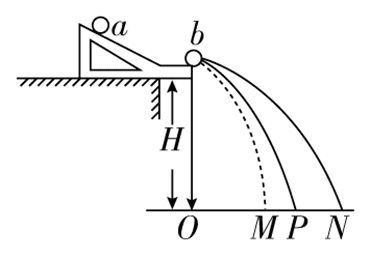在用如图所示的装置做“探究碰撞中的不变量”实验时，入射球 a 的质量为 m1，被碰球 b 的质量为 m2，小球的半径为 r，各小球的落地点如图所示，下列关于这个实验的说法中正确的是（    ）

A．入射球与被碰球最好采用大小相同、质量相同的小球

B．让入射球与被碰球连续 10 次相碰，每次都要使入射小球从斜槽上不同的位置滚下

C．要验证的表达式是 m1·ON = m1·OM + m2·OP

D．要验证的表达式是 m1·OP = m1·OM + m2·ON

【答案】

D

【解析】

8．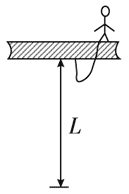如图所示，一高空作业的工人重为 600 N，系一条长为 L = 5 m 的安全带，若工人不慎跌落时安全带的缓冲时间 t = 1 s，则安全带受的冲力是多少？（g 取 10 m/s2

【答案】

1200 N，方向竖直向下

9．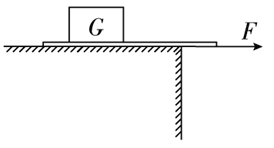如图所示，把重物 G 压在纸带上，用一水平力 F 缓慢拉动纸带，重物跟着纸带一起运动；若迅速拉动纸带，纸带将会从重物下抽出。对这种现象的解释正确的是（    ）

A．在缓慢拉动纸带时，纸带对重物的摩擦力大

B．在迅速拉动纸带时，纸带对重物的摩擦力小

C．在缓慢拉动纸带时，纸带对重物的冲量大

D．在迅速拉动纸带时，纸带对重物的冲量小

【答案】

CD

【解析】

10．为估算池中睡莲叶面承受雨滴撞击产生的平均压强，小明在雨天将一圆柱形水杯置于露台，测得 1 小时内杯中水位上升了 45 mm。查询得知，当时雨滴竖直下落的速度约为 12 m/s，据此估算该压强约为（设雨滴撞击睡莲后无反弹，不计雨滴重力，雨水的密度为 1 × 103 kg/m3）（     ）

A.0.15 Pa                 B．0.54 Pa              C．1.5 Pa                D．5.4 Pa

【答案】

A

【解析】

1 小时内雨的总质量 m = ρSh，对水列动量定理，向上为正方向，Ft = 0 − （− mv），再由压强公式 p = $$\frac{F}{S}$$，得 p = $$\frac{{\rho hv}}{t}$$，代入数据得 p = 0.15 Pa。A 选项正确。

11．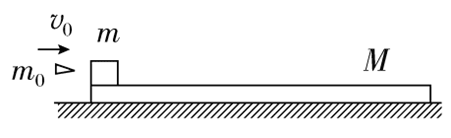如图所示，质量为 m = 245 g 的物块（可视为质点）放在质量为 M = 0.5 kg 的木板左端，足够长的木板静止在光滑水平面上，物块与木板间的动摩擦因数为 μ = 0.4。质量为 m0 = 5 g 的子弹以速度 v0 = 300 m/s 沿水平方向射入物块并留在其中（时间极短），g 取 10 m/s2。子弹射入后，求：

（1）物块相对木板滑行的时间；

（2）物块相对木板滑行的位移。

【答案】

（1）1 s

（2）3 m

【解析】

（1）子弹打入木块过程，由动量守恒定律得

m0v0 = （m0 + mv1

m0 + mv1 = （m0 + m + Mv2

－ μm0 + mgt = （m0 + m）（v2v1

（2）由能量守恒定律得

μm0 + mgd = $$\frac{1}{2}$$（m0 + mv12 − $$\frac{1}{2}$$（m0 + m + Mv22

12．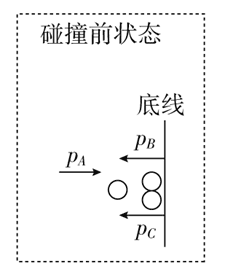如图所示，在橄榄球比赛中，一个 85 kg 的前锋队员以 5 m/s 的速度跑动，想穿越防守队员到底线触地得分。就在他刚要到底线时，迎面撞上了对方两名均为 65 kg 的队员，一个速度为 2 m/s，另一个速度为 4 m/s，然后他们就扭在了一起，则（    ）

A．他们碰撞后的共同速率是 0.2 m/s

B．碰撞后他们动量的方向仍向前

C．这名前锋能得分

D．这名前锋不能得分

【答案】

BC

【解析】

13．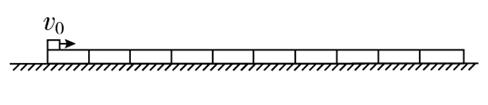如图所示，光滑水平地面上依次放置着质量 m = 0.08 kg 的 10 块完全相同的长直木板。一质量 M = 1.0 kg、大小可忽略的小铜块以初速度 v0 = 6.0 m/s 从长木板左侧滑上木板，当铜块滑离第一块木板时，速度大小为 v1 = 4.0 m/s。铜块最终停在第二块木板上。（取 g = 10 m/s2，结果保留两位有效数字）求 ：

（1）第一块木板的最终速度的大小；

（2）铜块的最终速度的大小。

【答案】

（1）2.5 m/s

（2）3.4 m/s

【解析】

（1）铜块和 10 个木板组成的系统水平方向不受外力，所以系统动量守恒，设铜块刚滑到第二块木板时，第一块木板的速度为 v2，由动量守恒得，

Mv0 = Mv1 + 10mv2

v2 = 2.5 m/s。

（2）由题可知铜块最终停在第二块木板上，设铜块的最终速度为 v3，由动量守恒得：Mv1 + 9mv2 = （M + 9mv3

v3 = 3.4 m/s。

14．两个小球 A、B 在光滑水平面上沿同一直线运动，其动量大小分别为 5 kg·m/s 和 7 kg·m/s，发生碰撞后小球 B 的动量大小变为10 kg·m/s，由此可知：两小球的质量之比可能为（    ）

A．$$\frac{{{m_{\rm{A}}}}}{{{m_{\rm{B}}}}}$$ = 1              B．$$\frac{{{m_{\rm{A}}}}}{{{m_{\rm{B}}}}}$$ = $$\frac{1}{2}$$             C．$$\frac{{{m_{\rm{A}}}}}{{{m_{\rm{B}}}}}$$ = $$\frac{1}{5}$$            D．$$\frac{{{m_{\rm{A}}}}}{{{m_{\rm{B}}}}}$$ = $$\frac{1}{10}$$

【答案】

C

15．在光滑水平面上，动能 Ek0，动量的大小为 p0 的小钢球 1 与静止小钢球 2 发生碰撞，碰撞前后球 1 的运动方向相反，将碰撞后球 1 的动能和动量大小分别记为 Ek1p1，球 2 的动能和动量的大小分别记为 Ek2p2，则必有（    ）

A．Ek1 < Ek0                   B．p1 < p0                       C．Ek2 > Ek0                 D．p2 > p0

【答案】

ABD

【解析】

16．光滑水平轨道上有三个木块 A、B、C，质量分别为 mA = 3mmB = mC = m，开始时 B、C 均静止，A 以初速度 v0 向右运动，A 与 B 碰撞后分开，B 又与 C 发生碰撞并粘在一起，此后 A 与 B 间的距离保持不变。求 B 与 C 碰撞前 B 的速度大小。

【答案】

$$\frac{6}{5}$$v0

【解析】

vA = v

vB = $$\frac{6}{5}$$ v0

17．载人气球静止于高 h 的空中，气球的质量为 M，人的质量为 m，若人沿绳梯滑至地面，则绳梯至少为多长？

【答案】

$$\frac{{M + m}}{M}$$ h

【解析】

2006 - 2023，推荐分辨率1024*768以上，推荐浏览器Chrome、Edge等现代浏览器，截止2021年12月5日的访问次数：1872万9823 站长邮箱